# sparrpowR: Power Analysis to Detect Spatial Relative Risk Clusters

#### 2021-06-28

``````loadedPackages <- c("broom", "geojsonio", "ggmap", "ggplot2", "grDevices", "maptools", "raster", "rgdal", "rgeos", "sf", "sp", "sparrpowR", "spatstat.geom", "tibble")
set.seed(1234) # for reproducibility``````

Import data from Open Data DC website.

``````# Washington, D.C. boundary
dc <- geojsonio::geojson_read(gis_path1,  what = "sp")

# American Community Survey 2018 Census Tracts
gis_path2 <- "https://opendata.arcgis.com/datasets/faea4d66e7134e57bf8566197f25b3a8_0.geojson"
census <- geojsonio::geojson_read(gis_path2,  what = "sp")``````

We want to create a realistic boundary (i.e., polygon) of our study area. We are going to spatially clip our DC boundary by the census tracts in an attempt to remove major bodies of water where people do not reside.

``````clipwin <- maptools::unionSpatialPolygons(census, IDs = rep(1, length(census)))
dcc <- rgeos::gIntersection(dc, clipwin, byid = TRUE)
# Plot
sp::plot(dc, main = "DC Boundary")
sp::plot(census,  main = "American Community Survey\n2018")
sp::plot(dcc, main = "Clipped Boundary")``````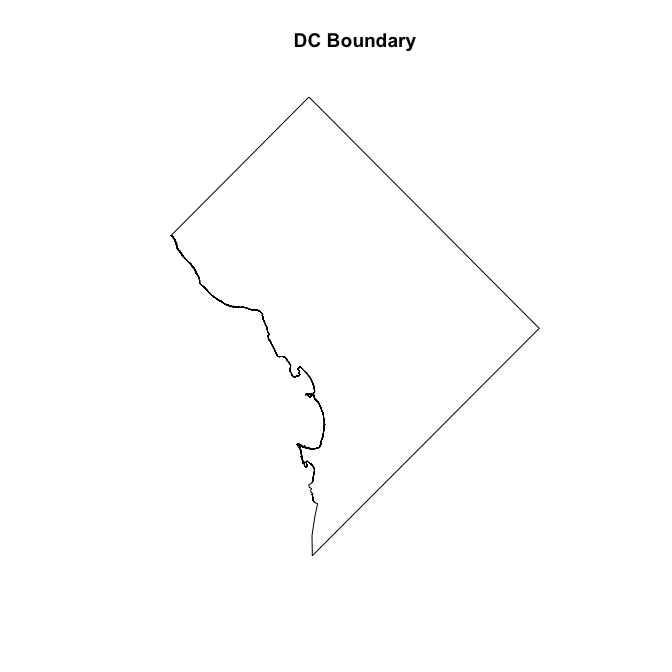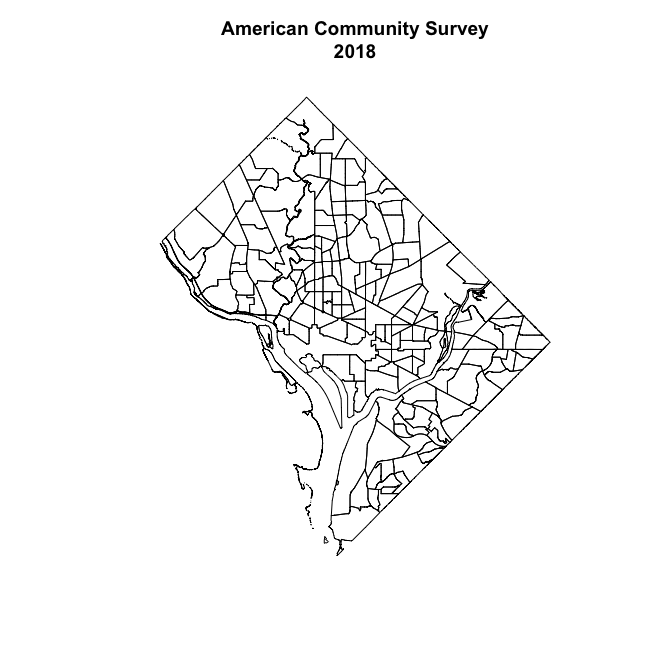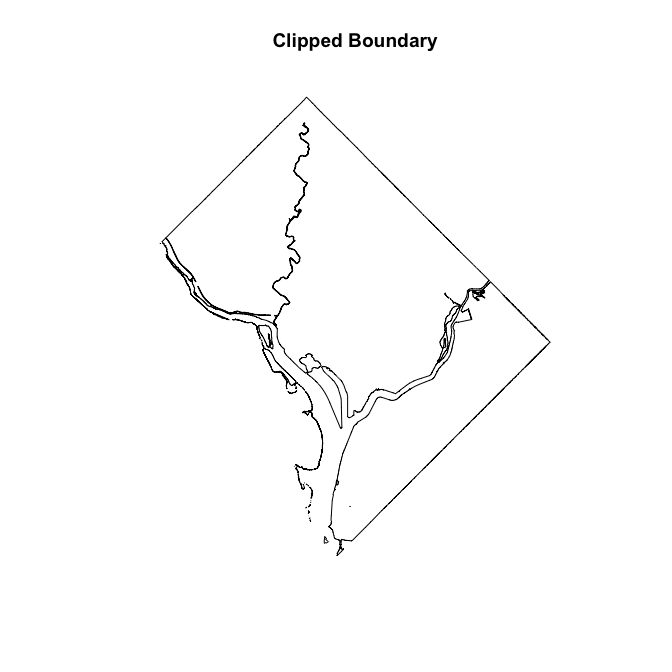Our developed method, sparrpowR, relies on the spatstat package suite to simulate data, which assumes point locations are on a planar (i.e., flat) surface. Our boundary is made up of geographical coordinates on Earth (i.e., a sphere), so we need to flatten our boundary by spatially projecting it with an appropriate spatial reference system (SRS). For the District of Columbia, we are going to use the World Geodetic System 1984 (WGS84) Universal Transverse Mercator (UTM) Zone 18N EPSG:32619. We then convert the boundary into a `owin` object required by the spatstat.geom package.

``````dcp <- sf::st_transform(sf::st_as_sf(dcc), crs = st_crs(32618))
dco <- spatstat.geom::as.owin(sf::st_geometry(dcp))``````

In this hypothetical example, we want to estimate the local power of detecting a spatial case cluster relative to control locations. Study participants that tested positive for a disease (i.e., cases) are hypothesized to be located in a circular area around the Navy Yard, an Environmental Protection Agency (EPA) Superfund Site (see the success story).

``````navy <- data.frame(lon = 326414.70444451, lat = 4304571.1539442)
spf <- sp::SpatialPoints(coords = navy, proj4string = sp::CRS(SRS_string = "EPSG:32618"))
# Plot
sp::plot(dcp, main = "Location of Hypothetical\nDisease Cluster")
sp::plot(spf, col = "magenta", add = T, pch = 4, cex = 2)
legend("bottom", xpd = T, y.intersp = -1.5, legend = c("Navy Yard"), col = "magenta", pch = 4, cex = 1, bty = "n")``````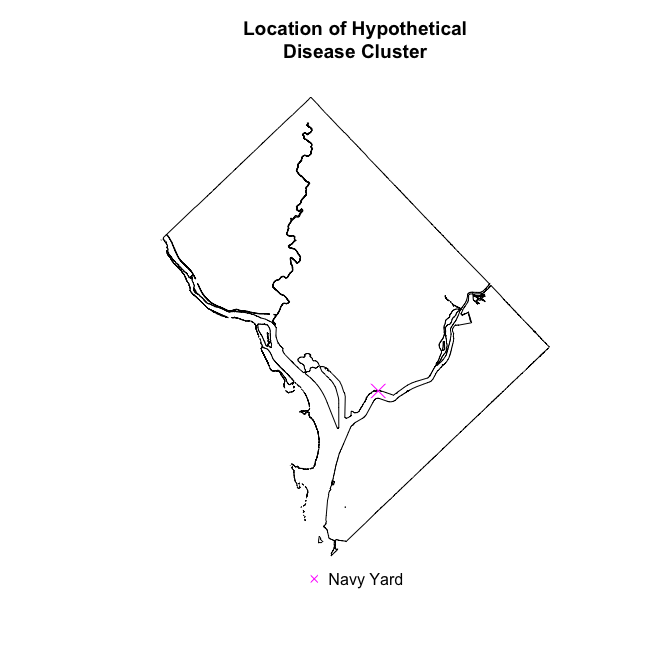We will assume that approximately 50 cases (e.g., `n_case = 50`) were clustered around the center of the Navy Yard (e.g., `samp_case = "MVN"`) with more cases near the center and fewer cases about 1 kilometers away (e.g., `s_case = 1000`).

If we were to conduct a study, where would we be sufficiently statistically powered to detect this spatial case cluster? To answer this question we will randomly sample approximately 950 participants (e.g., `n_conrol = 950` or 5% disease prevalence) around the Navy Yard (e.g., `samp_control = "MVN"`) sampling more participants near the center and fewer participants about 2 kilometers away (e.g., `s_control = 2000`). These participants would test negative for a disease (i.e., controls) were we to conduct a study. We can then resample control locations iteratively, as if we conducted the same study multiple times (e.g., `sim_total = 100`). We can conclude that we are sufficiently powered to detect a spatial clustering in areas where a statistically significant spatial case cluster was located in at least 80% (e.g., `p_thresh = 0.8`) of these theoretical studies. The `spatial_power()` function calculates both a one-tailed, lower tailed hypothesis (i.e., case clustering only) and a two-tailed hypothesis (i.e., case and control clustering). Use the `cascon` argument in the `spatial_plots()` function to plot either test.

``````start_time <- Sys.time() # record start time
sim_power <- spatial_power(x_case = navy[], y_case = navy[], # center of cluster
x_control = navy[], y_control = navy[], # center of cluster
n_case = 50, n_control = 950, # sample size of case/control
samp_case = "MVN", samp_control = "MVN", # samplers
s_case = 1000, s_control = 2000, # approximate size of clusters
alpha = 0.05, # critical p-value
sim_total = 100, # number of iterations
win = dco, # study area
resolution = 100, # number gridded knots on x-axis
edge = "diggle", # correct for edge effects
h0 = NULL, # automatically select bandwidth for each iteration
verbose = FALSE) # no printout
end_time <- Sys.time() # record end time
time_srr <- end_time - start_time # Calculate run time``````

The process above took about 18.9 minutes to run. Of the 100 iterations, we simulated 40 case locations and an average 766 (SD: 11.61) control locations for an average prevalence of 5.22% (SD: 0.08%). The average bandwidth for the statistic was 0.8 kilometers (SD: 0.01). Fewer case locations and controls locations were simulated than specified in the inputs due to being placed outside of our study window (i.e., Maryland, Virginia, or in the water features around the District of Columbia). Users can modify their inputs to achieve the correct number of cases and controls in their output.

We plot the statistical power for a one-tailed, lower-tail hypothesis (`cascon = FALSE`) at `alpha = 0.05` using the `spatial_plots()` function.

``````cols <- c("deepskyblue", "springgreen", "red", "navyblue") # colors for plots
chars <- c(4,5) # symbols for point-locations
sizes <- c(0.5,0.5) # size of point-locations
p_thresh <- 0.8 # 80% of iterations with statistically significant results

## Data Visualization of Input and Power
spatial_plots(input = sim_power, # use output of above simulation
p_thresh = p_thresh, # power cut-off
cascon = FALSE, # one-tail, lower tail hypothesis test (i.e., case clustering)
plot_pts = TRUE, # display the points in the second plot
chars = chars, # case, control
sizes = sizes, # case, control
cols = cols) # colors of plot``````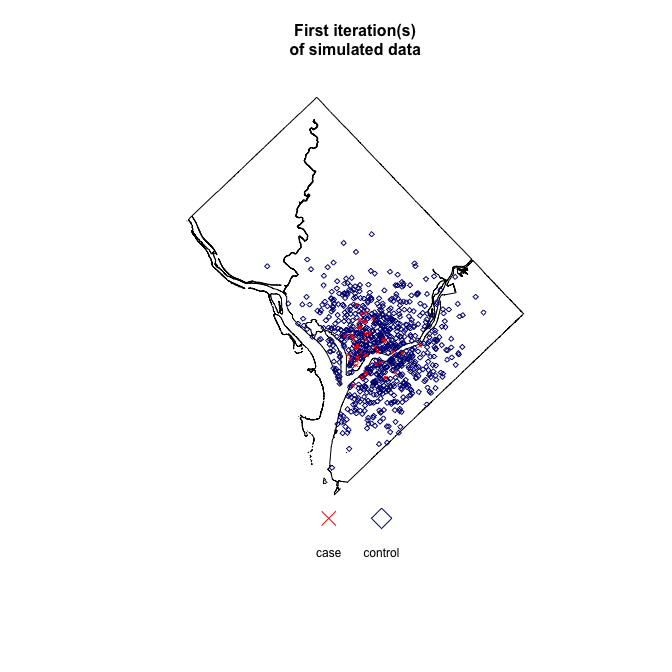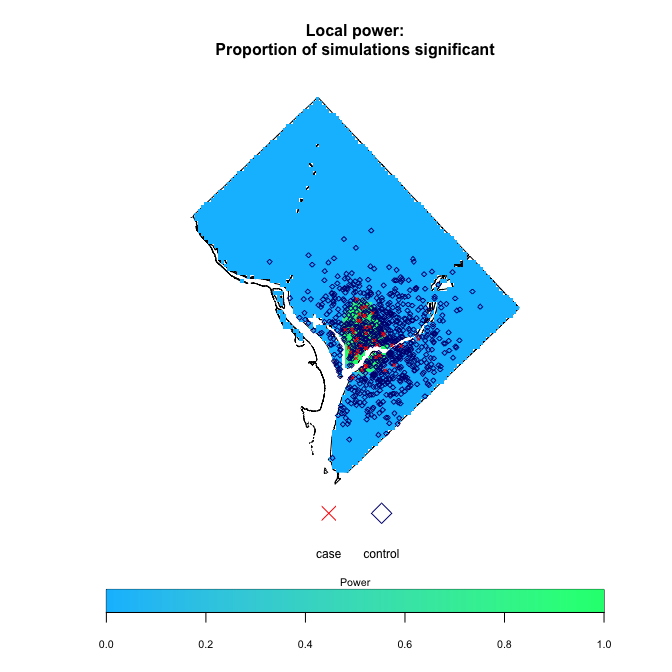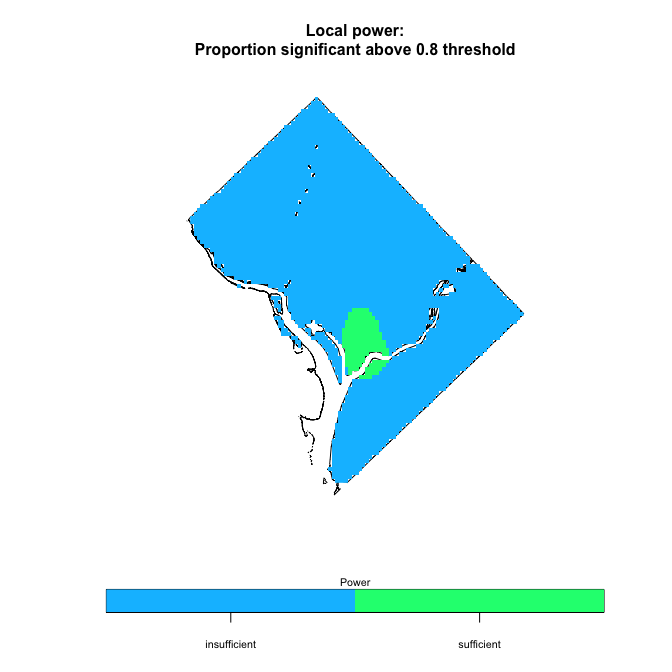Now, lets overlay our results on top of a basemap. Here, we will use an open-source map from Stamen, that is unprojected in WGS84. We extract the rectangular box (i.e., bounding box) surrounding our polygon boundary of the District of Columbia (WGS84).

``````dcbb <- sf::st_bbox(sf::st_buffer(sf::st_as_sf(dc), dist = 0.015))
dcbbm <- matrix(dcbb, nrow = 2)
base_map <- ggmap::get_map(location = dcbbm, maptype = "toner", source = "stamen")``````

Prepare the points from the first simulation for plotting in ggplot2 suite and prepare the original boundary for plotting in ggplot2 suite.

``````sim_pts <- sim_power\$sim  # extract points from first iteration
sim_pts <- maptools::as.SpatialPointsDataFrame.ppp(sim_pts) # convert to spatial data frame
raster::crs(sim_pts) <- sp::CRS(SRS_string = "EPSG:32618") # set initial projection
sim_pts_wgs84 <-  sp::spTransform(sim_pts, CRSobj = sp::CRS(SRS_string = "EPSG:4326")) # project to basemap
sim_pts_df <- tibble::tibble(data.frame(sim_pts_wgs84)) # convert to tidy data frame

# Original boundary
dc_df <- broom::tidy(dcc) # convert to a tidy dataframe
dcc\$polyID <- sapply(slot(dcc, "polygons"), function(x) slot(x, "ID")) # preserve polygon id for merge
dc_df <- merge(dc_df, dcc, by.x = "id", by.y="polyID") # merge data``````

Prepare the raster from the simulation for plotting in ggplot2 suite.

``````pvalprop <- tibble::tibble(x = sim_power\$rx, y = sim_power\$ry,
z = sim_power\$pval_prop_cas) # extract proportion significant
lrr_narm <- na.omit(pvalprop) # remove NAs
sp::coordinates(lrr_narm) <- ~ x + y # coordinates
gridded(lrr_narm) <- TRUE # gridded
pvalprop_raster <- raster::raster(lrr_narm) # convert to raster
rm(pvalprop, lrr_narm) # conserve memory
raster::crs(pvalprop_raster) <- raster::crs(dcp) # set output project (UTM 18N)
pvalprop_raster <- raster::projectRaster(pvalprop_raster, crs = raster::crs(dc)) # unproject (WGS84)
rtp <- raster::rasterToPolygons(pvalprop_raster) # convert to polygons
rtp@data\$id <- 1:nrow(rtp@data)   # add id column for join
rtpFort <- broom::tidy(rtp, data = rtp@data) # convert to tibble
rtpFortMer <- merge(rtpFort, rtp@data, by.x = 'id', by.y = 'id')  # join data
rampcols <- grDevices::colorRampPalette(colors = c(cols, cols), space="Lab")(length(raster::values(pvalprop_raster))) # set colorramp``````

Plot local power as a continuous outcome with point-locations using the ggplot2 suite.

``````ggmap::ggmap(base_map) + # basemap
ggplot2::geom_polygon(data = dc_df, # original boundary
ggplot2::aes(x = long, y = lat, group = group),
fill = "transparent",
colour = "black") +
ggplot2::geom_polygon(data = rtpFortMer, # output raster as polygons
ggplot2::aes(x = long, y = lat, group = group, fill = z),
size = 0,
alpha = 0.5) +
ggplot2::scale_fill_gradientn(colours = rampcols) + # colors for polygons
ggplot2::geom_point(data = sim_pts_df, # simulated point-locations
ggplot2::aes(x = mx, y = my, color = marks, shape = marks),
alpha = 0.8) +
ggplot2::scale_color_manual(values = cols[3:4]) + # fill of point-locations
ggplot2::scale_shape_manual(values = chars) + # shape of point-locations
ggplot2::labs(x = "", y = "", fill = "Power", color = "", shape = "") # legend labels``````